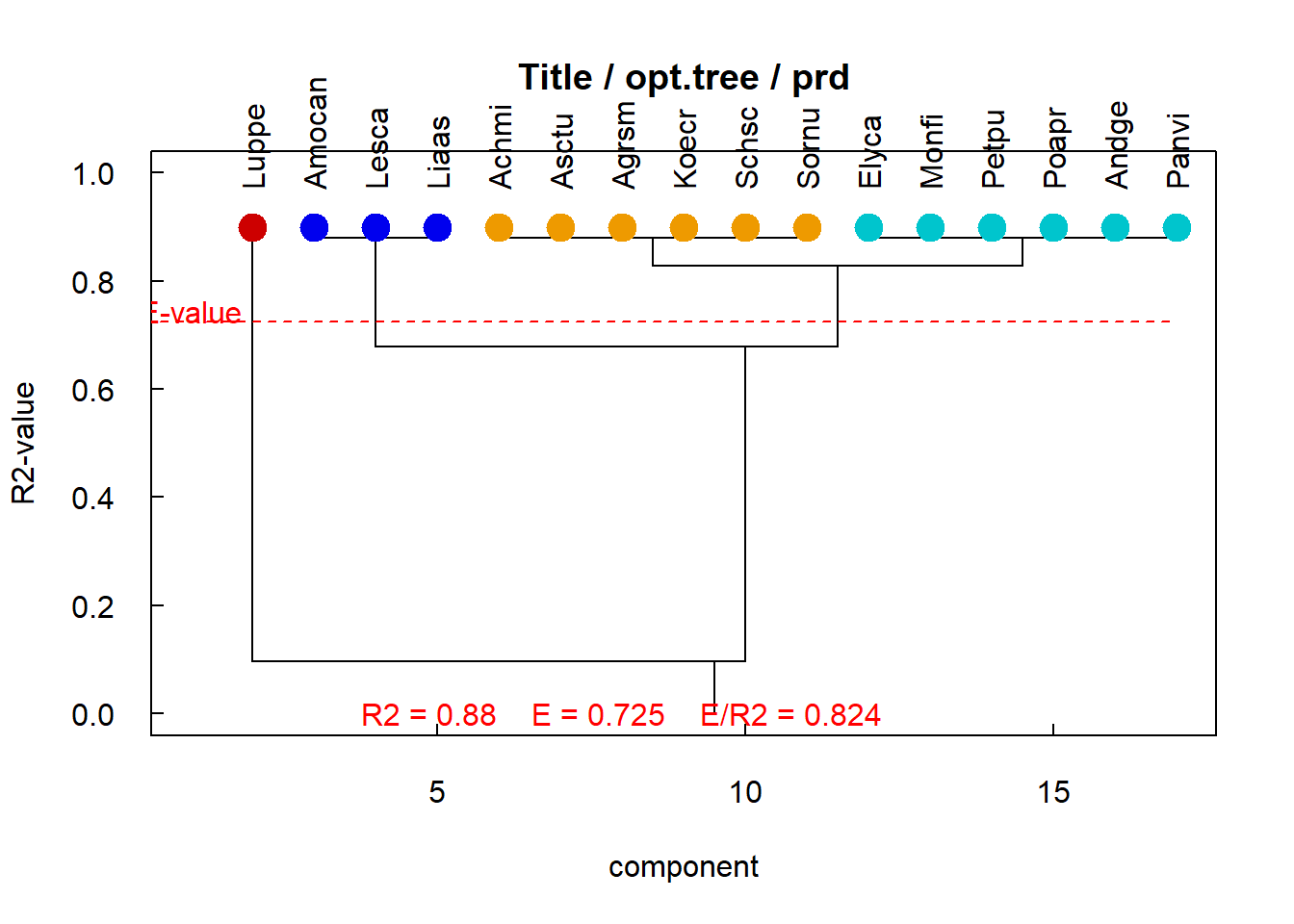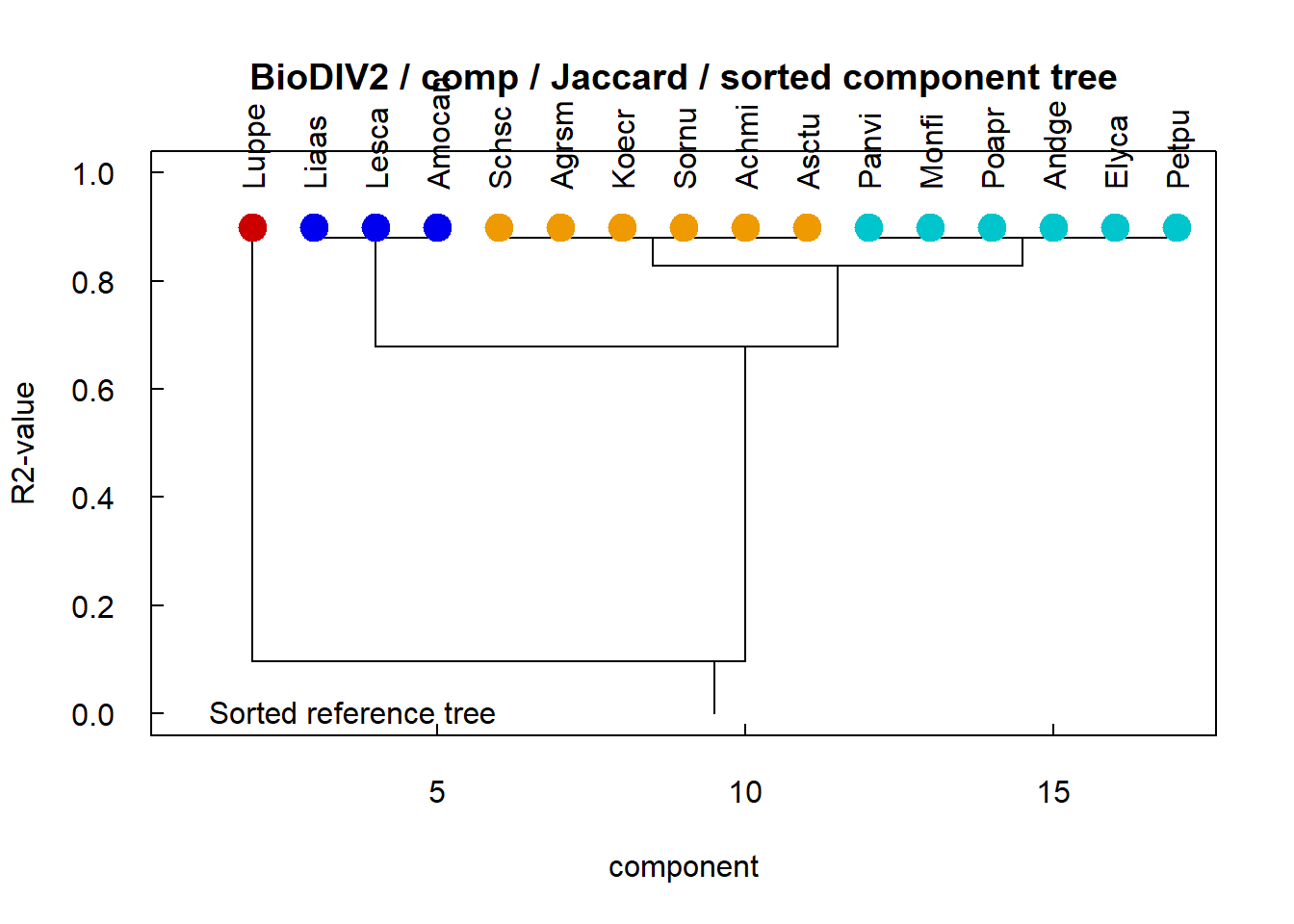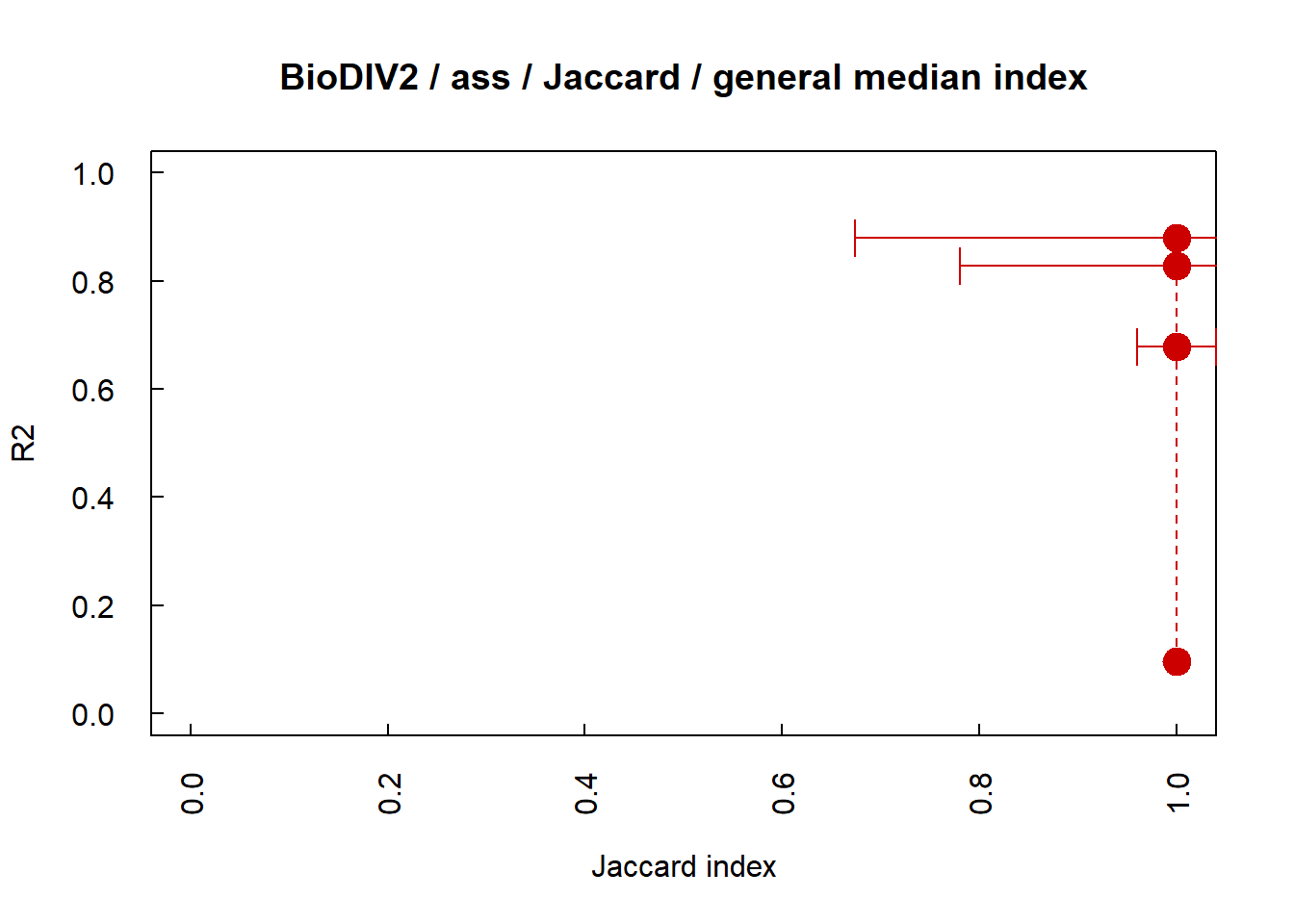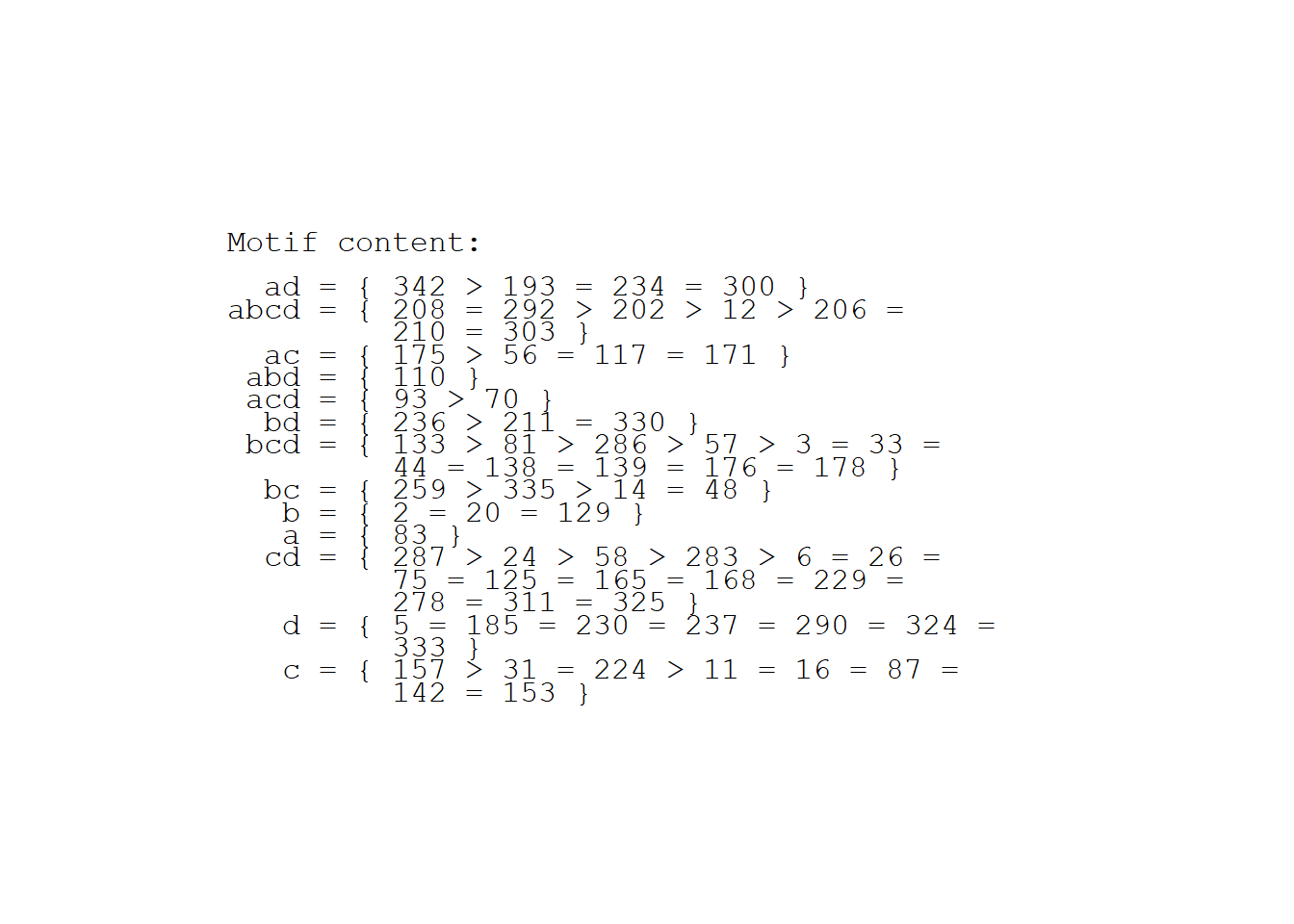# Testing significance of different variables with functClust

## Are all components or observations equally important?

Functional clustering aims to identify the role played by each component belonging to an interactive system on the genesis of a collective, system-specific performance. It needs the observation of a collection of assemblages of components of different elemental composition, of which collective, system-specific performances are observed. A functional clustering groups the components of the interactive system on the basis of their effects on the system-specific performance. The method hierarchises de facto the functional groups: the first division explains most of the observed variance, and the subsequent divisions explain a smaller and smaller part of the observed variance.

Functional clustering thus leads to ask the following questions: are some components that belong to each functional group more efficient than others? How can component efficiency be assessed? Is it possible to priorize components for their effect on system performance? Similar questions can be raised about relative importance of different observations, that is different observed component assemblages of the collection, or of different observed performances in the case of repeated observations of the performance, or the observations of different properties carried out by the interactive system.

The function ftest answer to the questions. The method is based on removing one element of the dataset and evaluating the perturbation induced on functional clustering. The removed element can be a system component, a component assemblage, or an assemblage performance. The induced perturbation is evaluated by comparing the clustering tree obtained with removing the element, to the reference clustering tree obtained with the complete dataset. Different indices are computed, using the R-package clusterCrit (Package “clusterCrit”: Clustering Indices, by Bernard Desgraupes, University of Paris Ouest - Lab Modal’X). The indices are: Precision index and Recall index, or indices proposed by different authors as Czekanowski_Dice index, Folkes_Mallows index, Jaccard index, Kulczynski index, Rogers_Tanimoto index, Russel_Rao index, and both Sokal_Sneath1 and Sokal_Sneath2 indices.

## The function ftest and its options

The function ftest needs first that the function fclust is run for computing the reference clustering tree obtained with the complete dataset. The object returned by fclust is noted fres. The options of ftest are:

• opt.var determines the variable to treat. The option can be “components”, “assemblages” or “performances”.

• opt.nbMax determines the tree level to be reached. The function ftest is very time-consuming since it is based on the repeat of functional clusterings. Then, only the validated lowest part of the tree is interesting, from trunk (tree-level = 1) until the optimum level fres$nbOpt. Beyond the optimum level fres$nbOpt, the clustering does not bring any supplementary information. The computation therefore deserves to be stopped at this tree level. In ftest, the option opt.nbMax = fres\$nbOpt by default. The option opt.nbMax allows the user to change the stop tree-level, more or less.

• opt.R2 determines if the user needs to compute other statistiques than clustering indices. If opt.R2 = TRUE, each primary tree is validated and the vectors of coefficient of determination R2 and efficiency E are computed.

• opt.plot determines if the user wish to follow the computation by plotting the resulting tree at each element removing. If option opt.plot = TRUE, the resulting tree is plotted at each element removing. The removed element is indicated on the tree plot.

The function ftest returns an object rtest, which consists on a list of matrices, each matrix containing the results for a given clustering index, and R2 and E if opt.R2 was checked.

## The function ftest_plot

The function ftest_plot first needs the object fres generated by the function fclust, and the object rtest generated by the function ftest. The options of ftest_plot are:

• opt.crit determines the indices to be plotted. The option opt.crit is a list or a vector of characters choisen among “Czekanowski_Dice”, “Folkes_Mallows”, “Jaccard”, “Kulczynski”, “Precision”, “Rand”, “Recall”, “Rogers_Tanimoto”, “Russel_Rao”, “Sokal_Sneath1” and “Sokal_Sneath2”.

• opt.var determines the variable to plot. The option can be “components”, “assemblages” or “performances”.

fclust_plot(fres = CedarCreek.2004.2006.res, opt.tree = "prd")

ftest_plot(fres = CedarCreek.2004.2006.res,
rtest = CedarCreek.2004.2006.test.components,
main = "BioDIV2",
opt.var = "comp", opt.crit = "Jaccard", opt.comp = "sorted.tree")Raw tree on left, Tree with components sorted by decreasing effect on right

The graph on the left is the raw tree, directly obtained with the function fclust. On the right, the components of the tree are sorted by their decreasing effect of Jaccard index when they are one by one removed from dataset. For instance, within the component cluster “b” (in blue), the effect induced by species on ecosystem biomass can be sorted as: “Liass” > “Lesca” > “Amocan”.

ftest_plot(fres = CedarCreek.2004.2006.res,
rtest = CedarCreek.2004.2006.test.assemblages,
main = "BioDIV2", opt.var = "assemblages")Raw tree on left, Tree with components sorted by decreasing effect on right

The graph shows the mean Jaccard index when each assemblage is one by one removed from dataset. And the text indicates the assemblages sorted by decreasing effect within each assembly motif on the functional clustering.
For instance, the assembly motif ad has the highest mean performance. The effect induced by removing assemblage can be sorted as: “plot 193” > “234” > “300” > “342”.

## The functions ftest_write and ftest_read

The functions ftest_write and ftest_read allow to save and load, respectively, the results generated by the function ftest.

## Note

Note that some computations are time-consuming. To facilitate the monitoring of the smooth running of the computations, informations are written on the Console and graphs are drawn on the Plots panel. The writting are enable or disable by the “verbose” option.

getOption("verbose")
#>  FALSE
options(verbose = FALSE)